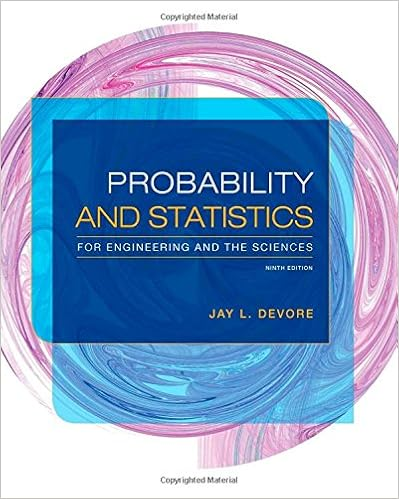# Follows from eq364 that the required condition of

• Notes
• rjgcabrera
• 27
• 100% (1) 1 out of 1 people found this document helpful

This preview shows page 12 - 14 out of 27 pages.

##### We have textbook solutions for you!
The document you are viewing contains questions related to this textbook.The document you are viewing contains questions related to this textbook.
Chapter 1 / Exercise 8
Probability and Statistics for Engineering and the Sciences
DevoreExpert Verified
follows from Eq.3.6.4 that the required condition of minimum power occurs when (3.6.5) which is usually referred to as the "cube law," or as "Murray's law" after its first author. The cube law has been used for many years and has shown considerable presence in the cardiovascular system [5-14]. It is important to recall that it rests on two important assumptions: (i) that the flow under consideration is steady fully developed Poiseuille flow, and (ii) that the optimality criterion being used is that of minimizing the total rate of energy expenditure for dynamic and metabolic purposes. Other laws have been considered by a number of authors to address observed departures or scatter away from the cube law [15-17]. It has been found, for example, that in the aorta and its first generation of major (l ) radius =a pumping power = Hs t SAME FLOW RATE l radius =0/2 (J ) pumping power = 16 Hs FIGURE 3.6.1. Pumping power required to maintain steady fully developed Poiseuille flow. If the radius of a tube is halved, the pumping power required to drive the same flow rate through it increases by a factor of 16 (1600%). Con- versely, if the radius of a tube is doubled, only about 6% of the power is required to drive the same flow rate through it.
##### We have textbook solutions for you!
The document you are viewing contains questions related to this textbook.The document you are viewing contains questions related to this textbook.
Chapter 1 / Exercise 8
Probability and Statistics for Engineering and the Sciences
DevoreExpert Verified
3.7 Arterial Bifurcation 51 branches, a "square law" in which the flow rate is proportional to a 2 may be more appropriate than the cube law. At more peripheral regions of the arterial tree, however, measured data suggest that the cube law eventually prevails, even if with considerable scatter. Because in Poiseuille flow the shear stress on the tube wall is proportional to the ratio qja 3 (Eq.3.4.7), the cube law is consistent with constant shear rate in the vascular system. That is, at higher levels of the arterial tree, as the diameters of vessel segments become smaller, flow rates within them also become smaller but in accordance with the cube law, leaving the shear stress on vessel walls unchanged. Because the range of diameters in the arterial tree extends over three to four orders of magnitude, any departure from this precarious design would lead to a range of shear stress of many orders of magnitude. If the flow rate varies in accordance with a square law (q ex: a 2 ) or a quartic law (q ex: a 4 ), for example, the shear stress on the vessel walls would be proportional to 1ja or to a, respectively. Thus as a varies by three to four orders of magnitude, so will the shear stress, and in the case of the square law the higher shear will occur in the smaller vessels. Because the lumen of vessels of all sizes are lined with essentially the same type of endothelial tissue, these scenarios seem unlikely on theoretical grounds. Thus the form of the shear stress in Poiseuille flow provides a strong theoretical support for the cube law, on grounds that are quite different from those on which the law was originally based.
•••